# Transforms¶

`transform`(mode=None)

Sets whether shapes are transformed along their centerpoint or (0,0).

The mode parameter can be CORNER (default) or CENTER.

It sets the registration point – the offset for `rotate()`, `scale()` and `skew()` commands. By default, primitives, text, and images rotate around their own centerpoints. But if you call transform() with CORNER as its mode parameter, transformations will be applied relative to the canvas top left corner (its “origin point”) instead.

See the examples in `translate()`, `rotate()`, `scale()` and `skew()` to see how the transform mode affects the result.

`translate`(xt, yt)

Specifies the amount to move the canvas origin point.

Once called, all commands following translate() are repositioned, which makes translate() useful for positioning whole compositions of multiple elements.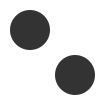```fill(0.2)
oval(10, 10, 40, 40)
translate(45, 45)
oval(10, 10, 40, 40)
```
`rotate`(degrees=0, radians=0)

Rotates all subsequent drawing commands.

The default unit is degrees; radians can be used with `rotate(radians=PI)`.

This command works incrementally: if you call `rotate(30)`, and later on call `rotate(60)`, all commands following that second rotate() will be rotated 90° (30+60).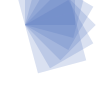```fill('#4a69bd', 0.2)
translate(25, 25)
for i in range(7):
rotate(15)
rect(0, 0, 50, 50)
```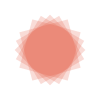```fill('#e55039', 0.2)
transform(CENTER)
for i in range(5):
rotate(15)
rect(25, 25, 50, 50)
```
`scale`(x=1, y=None)

Increases, decreases, or streches the size of all subsequent drawing commands.

The first parameter sets the horizontal scale and the optional second parameter the vertical scale. You can also call scale() with a single parameter that sets both the horizontal and vertical scale. Scale values are specified as floating-point (decimal) numbers with 1.0 corresponding to 100%.

This command works incrementally: if you call `scale(0.5)`, and later on call `scale(0.2)`, all subsequent drawing commands will be sized to 10% (0.2 of 0.5).```fill('#78e08f', 0.2)
translate(25,25)
for i in range(7):
rect(0, 0, 50, 50)
scale(.8)
``````fill('#60a3bc', 0.2)
transform(CENTER)
for i in range(7):
rect(25, 25, 50, 50)
scale(.8)
```
`skew`(x=1, y=0)

Slants the direction of all subsequent drawing commands.

The first parameter sets the horizontal skew. The second parameter is optional and sets the vertical skew.

This command works incrementally: if you call `skew(10)`, and later on call `skew(20)`, all subsequent drawing commands will be skewed by 30° (10+20).```fill('#82ccdd', 0.2)
translate(5, 25)
for i in range(7):
rect(0, 0, 50, 50)
skew(.2, 0)
``````fill('#e58e26', 0.2)
transform(CENTER)
for i in range(7):
rect(25, 25, 50, 50)
skew(.2, 0)
```
`push`()

Saves the current transform state.

The push() function, along with its companion pop(), allows for “saving” a transform state. All transformations, such as rotate() and skew(), defined between push() and pop() will stop being applied after pop() is called.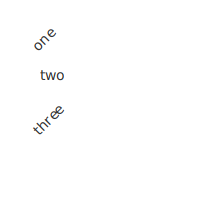```fill(0.2)
fontsize(14)
transform(CENTER)
rotate(45)
text("one", 40, 40)

push()
rotate(-45)
text("two", 40, 80)
pop()

text("three", 40, 120)
```
`pop`()

Restores the saved transform state.

This command is meant to be used after push(). It “loads” the transform state that was set before the call to push().

`reset`()

Resets the transform state to its default values.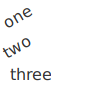```transform(CENTER)
rotate(30)
text("one", 10, 20)
text("two", 10, 50)
reset()
text("three", 10, 80)
```